# Electrical Properties

Organic molecules with π-bonding and wave function overlap behave as semiconductors. The degree of conductivity depends on the bandgap.

## Contents

### Bandgap and conductivity

In order to have a net electrical current electrons must jump from completely filled levels to empty levels across the bandgap. If the bandgap (Eg) is large, upon applying an external electric field at room temperature, there will be few electrons that have the necessary energy to jump from the valence band to the conduction band. Thermal energy can in some cases provide enough energy to elevate an electron into the conduction band.

Thermal Energy is  :

at 300K: kT ~ 0.025 eV (1/40 eV)
~ 0.6 kcal/mol
~ 2.5 kJ/mol

For Eg >=2eV is considered an insulator. Very few electrons can jump the bandgap.

σRT is typically;
<= 10-10 Ω -1 cm-1
<= 10-10 S/ cm

The best insulators go down to 10-16 S/cm

0 < Eg ≤ 2eV: semiconductor

10-10 ≤ σRT ≤ 102 S/cm
polyacetylene Eg ~ 1.5 eV
Si Eg ~ 1.1 eV

for Eg → 0: metal

σRT ≥ 102 S/cm

Electrical conductivities vary over 25 orders of magnitude from the best insulator to the best conductor. A single crystal of copper at room temperature has a conductivity of 6 x 105 S/cm

σRT Cu ~ 6 x 105 S/cm
Ag, Au ~ 106 S/cm

### Conductivity Defined

Electrical conductivity (σ) can be described with 3 terms.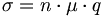$\sigma = n \cdot \mu \cdot q\,\!$

Where

n = density of charge carriers (cm-3)
μ = mobility of charge carrier (cm 2/Vs)
q= charge (Coulombs, Cb)

To have conduction there must be charge carriers. For example transpolyacetylene at 0°K has a valence band that is completely full and a conduction band that is completely empty. There are electrons but they can not participate in the charge transfer process. If an electron is elevated to the excited state it enters the conduction band (LUMO level) becomes a charge carrier. If you then apply an electric field the charges need to be able to move in order for there to be a current. If the bond between the ethylene subunits were much longer there would be no overlap between their atomic orbitals and there would be zero electronic coupling between the π orbitals. The mobility of the charge would be zero.

In fact, the bond is only 1.45 Å and there is a bonding and antibonding level that allows for electronic coupling between subunits. Energy splitting of these levels is a measure of the strength of electronic coupling between the subunits. In transpolyacetyline the bandwidth of the valence and conduction bands is about 5eV, which corresponds to an energy splitting electronic coupling (t) of 2t. Organic semiconductors may have an electronic coupling of t= 2.5eV, which is quite large.

Charge mobility is the average speed of diffusion of the charge carriers (cm/s) as a function of applied electric field (V/cm). Organic transistors or an electronic device made from organic materials must have good mobility of the charge carriers. There are many papers in the literature which characterize the charge mobility of organic compounds. A good mobility is 1 or larger.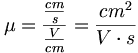$\mu = \frac {\frac {cm} {s}} {\frac {V} {cm}} = \frac {cm^2} {V \cdot s} \,\!$

We can use dimensional analysis to make sure that all the units reflect the components of the equations.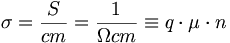$\sigma = \frac {S} {cm} = \frac {1} {\Omega cm} \equiv q \cdot \mu \cdot n\,\!$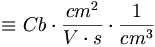$\equiv Cb \cdot \frac {cm^2} {V \cdot s} \cdot \frac {1} {cm^3}\,\!$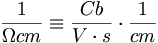$\frac {1} {\Omega cm } \equiv \frac{ Cb} {V \cdot s} \cdot \frac {1} {cm}\,\!$

(R = V/i and Ω = V.s /Cb)

### Charge Factor

Usually the charge (q) is simply the electronic charge of an electron. When an ionic compound such as salt is dissolved in water it forms ions.

Solid salt is white. This is an indication that it is an insulator because it means that does not absorb light in the visible spectrum. The visible spectrum is 1.5eV – 3eV so as white material absorbs beyond the visible spectrum. If the first optical transition is beyond the visible, bandgap is larger than 3eV it is an insulator. If the crystal is perfect such as diamond you could have an optical bandgap of 9eV. Salt looks white because of multiple scattering from smaller crystals. But when you put sodium chloride in water it dissociates into ions. The ions are charge carriers. In the case of calcium sulfate each ion will have a charge that is twice as large (+2 or -2 charge). Thus some ions charge carrier will have charge multiples greater than 1.

### Temperature and mobility

At 0 ° Kelvin semiconductors and insulators there is a significant bandgap and no charge carriers. Increasing the temperature increases the chance for electrons to jump from the valence band to the conduction band. Charge carrier density rises exponentially. Charge mobility on the other hand goes down with higher temperature. However the increase in charge density with temperature is far less than the decrease in mobility with temperature so the there is a net increase in conductivity with increase temperature.